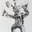Question-and-Answer Resource for the Building Energy Modeling Community
Get s tarted with the Help page

# HeatPump:WaterToWater:EIR models' curves report wrong valuesI'm using the water-to-water heat pump models HeatPump:WaterToWater:EIR:Heating and Cooling in simple hot and chilled water loops connected to a fancoil. The water temperatures and mass flow rates in both loop nodes look fine. I got good values for the outputs of the heat pump when I used the constant performance curves in the example file for this model. But when I have started using curves with values, I have started having a mess with the model results.

EDIT: From the results I detailed below, I think that the model is using the EIRFT curve to get both the "EIR-function of temperatures" and "EIR-function of PLR" modifiers. Then in the curve output variables I can only see the last action performed (to get the EIRFPLR modifier).

Then, if I prepare an EIRFT table with the PLR and Outlet hot water temperatures in the x axis, I can get the expected heat pump power consumption results.

Can anyone confirm this please? I am really interested in using this heat pump model.

The EIR modifier function of temperature curve (EIRFT)

The EIRFT curve should use the Outlet hot/chilled water temperature as "Input variable 1". However, when I look at the output variable "Performance Curve Input Variable 1 Value" for this curves, I'm not obtaining the expected value.

I think this curve is getting as input the PLR value instead. Although the water-to-water heat pump PLR is not available for reporting, the value I'm getting as "input variable 1" matches "PLR=Load Side Heat Transfer Rate / (Reference Capacity*CAPFT)".

The "Performance Curve Input Variable 2 Value" output works well, and the curve output is consistent with the reported input values.

The EIR modifier function of PLR curve (EIRFPLR)

I get 0 as both input and output value when I report the curve output variables. I make changes in this curve and the heat pump outputs (electric power and source side heat transfer rate) remain the same although the heat pump isn't working at full load (PLR<1).

The model equations

I couldn't find the model equations in the Engineering Reference Manual for this object. I guess they are the same as the EIR-based chiller model:

   Power consumption = CAPref* CAPFT * (1/COPref) * EIRFT * EIRFPLR * Cycling Ratio

Cycling Ratio = PLR / PLRmin    (I assumed PLRmin=1 because there is no input for PLRmin)


I couldn't actually check whether I'm getting consistent results, because the reported "EIRFPLR" output value is 0. However I have all the other values, so I tried to solve for it. The EIRFPLR value I found turned out to be exactly the expected EIRFT value for the correct input variables (not the reported ones).

Error with EIRFT table dimensions and transition version

Maybe this other issue is related. In version 9.1 this heat pump model accepted a "Table:TwoIndependentValues" object as EIRFT curve. In version 9.2, it doesn't accept the equivalent "Table:Lookup" object with two independent variables (although ...

edit retag close merge delete

Sort by » oldest newest most votedYou conclusion is correct " that the model is using the EIRFT curve to get both the "EIR-function of temperatures" and "EIR-function of PLR" modifiers." A new issue has been posted to fix this and there is some additional explanation there. See #7590.

Because the same curve is being evaluated (incorrectly) with the temperature variables first, and then with the plr, the output reporting for that curve will hold the inputs/results of the second invalid call.

Regarding the transition for Table:TwoIndependentValues, another user just posted an issue noting the same problem. See #7588.

more